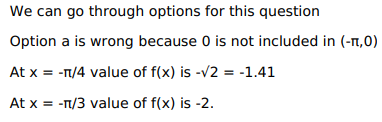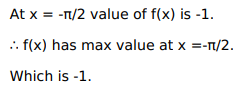# Solve this following

Question:

Mark $(\sqrt{)}$ against the correct answer in the following:

$f(\mathrm{x})=\operatorname{cosec} \mathrm{x}$ in $(-\pi, 0)$ has a maxima at

A. $x=0$

B. $x=\frac{-\pi}{4}$

C. $x=\frac{-\pi}{3}$

D. $\mathrm{x}=\frac{-\pi}{2}$

Solution: